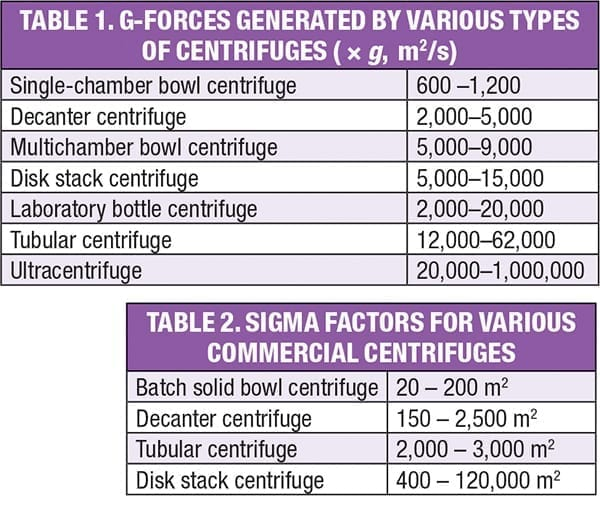Processing & Handling

# Facts at your Fingertips: Centrifuge Separation Capacity

| By Scott Jenkins

This one-page reference describes how to measure the capability of a given centrifuge to separate solids from a liquid phase.

## G-force

The centrifugal force developed by a centrifuge is expressed as a multiple of the force of gravity. This force, known as the G-force, is proportional to the distance from the axis of rotation and the square of the rotational speed, as shown in Equation (1).

G = 39.48 n 2 r / g                    (1)

Where:

G is the G force

is the rotational speed, revolutions per second (rev/s)

r  is the distance from the axis of rotation, cm

g  is acceleration due to gravity, which has a conventional standard value of 981 cm/s2, but depends on altitude

Equation (1) indicates the primary parameters available to designers to obtain the desired G-force — the diameter and rotor rotational speed. The G-force is not uniform throughout the centrifuge. The force is smallest near the axis of rotation, and then increases linearly in the radial direction. Typical G-forces developed by several types of centrifuges vary widely, ranging from a low of 600 × g for the single-chamber bowl centrifuge, to as high as 1,000,000 for the ultracentrifuge (Table 1). Values at the high end are sufficient to remove macromolecules, such as nucleic acids.## Sigma factor

While the G-force is an important characteristic of a centrifuge, it may not be a complete indicator of separation capability. This is because centrifuge performance is impacted not only by the applied force, but also by the area available for settled solids. That is, for a given applied G-force, a centrifuge with more settling area offers greater separation power.

A better measure of the separation capability of a centrifuge is its sigma factor, ∑, which is defined as the cross-sectional area of a gravity settling tank that would give the same separation performance. Values depend on centrifuge geometry and configuration (for instance, the size and number of settling surfaces), in addition to the G-force. For some types of centrifuges, equations for direct calculation of the sigma factor are given in engineering handbooks.

Typical sigma factors for several types of centrifuges are provided in Table 2. As with G-force, ∑ values vary widely, ranging from a low of 20 m2 for the batch solid bowl, up to 120,000 m2 for the disk-stack centrifuge. Although the decanter is highly versatile and widely used, as discussed below, its sigma factor is relatively low because of its relatively low settling area. Conversely, the disk stack centrifuge contains a large number of disks, providing substantial settling area, and in turn, a high sigma factor.

Please note that the sigma factors shown in Table 2 are only approximations; because of complex flow patterns within the centrifuge, actual performance may deviate by as much as 50% from the given values.

Sigma factors can be employed to size a production-scale centrifuge using pilot data obtained with the same type of centrifuge, according to the relationship shown in Equation (2):

Q 2 = ( Q 12) / ∑ 1                            (2)

Where: Q is the flowrate and ∑ is the sigma factor

Subscripts 1 and 2 refer to pilot and production scale, respectively. Equation (2) says that if an optimized throughput of Q1 is obtained with a pilot centrifuge having a sigma factor of ∑1, then a production unit with a sigma factor of ∑2 will be needed to obtain the desired production flowrate of Q2. Equation 2 can also be used to determine if the desired throughput can be achieved with an existing centrifuge with a known value of ∑2. That value and the pilot data are used to calculate Q2, which is then compared to the desired value to see if the centrifuge is suitable. However, because of the approximate nature of sigma factors, results obtained using this approach should be regarded as no more than rough estimates.

## Key parameters

Important parameters for centrifuge operations include the process objective (whether the solid is the valuable product or the liquid is valuable), the physical properties of the feed, the density difference between the solid material and the liquid phase, the particle size and shape and the viscosity of the liquid. The ease of separation increases with an increase in the density difference between the solids and the liquid, meaning less force is needed and a smaller centrifuge (or a centrifuge operated at a lower rotational speed) can be used to obtain a given throughput.

Smaller particles are more difficult to remove than larger ones, because in general, the sedimentation rate increases with the square of the particle diameter. The centrifuge design needs to be based on the smallest particle to be removed. However, not only is the particle size important, but also its shape. For example, flat, elongated particles tend to settle more slowly than spherical ones. In addition, solids tend to settle more slowly with increasing liquid viscosity, requiring a larger centrifuge or higher rotational speed to achieve the desired capacity.

Editor’s note: Material in this column comes from Gabelman, A., Beyond Gravity: Centrifugal Separations in CPI Operations, Chem. Eng., July 2016, pp. 52–59.